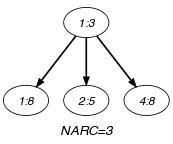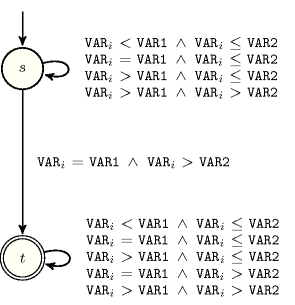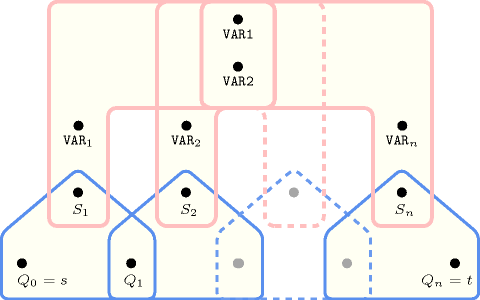## 5.264. minimum_greater_than

Origin

N. Beldiceanu

Constraint

$\mathrm{𝚖𝚒𝚗𝚒𝚖𝚞𝚖}_\mathrm{𝚐𝚛𝚎𝚊𝚝𝚎𝚛}_\mathrm{𝚝𝚑𝚊𝚗}\left(\mathrm{𝚅𝙰𝚁}\mathtt{1},\mathrm{𝚅𝙰𝚁}\mathtt{2},\mathrm{𝚅𝙰𝚁𝙸𝙰𝙱𝙻𝙴𝚂}\right)$

Arguments
 $\mathrm{𝚅𝙰𝚁}\mathtt{1}$ $\mathrm{𝚍𝚟𝚊𝚛}$ $\mathrm{𝚅𝙰𝚁}\mathtt{2}$ $\mathrm{𝚍𝚟𝚊𝚛}$ $\mathrm{𝚅𝙰𝚁𝙸𝙰𝙱𝙻𝙴𝚂}$ $\mathrm{𝚌𝚘𝚕𝚕𝚎𝚌𝚝𝚒𝚘𝚗}\left(\mathrm{𝚟𝚊𝚛}-\mathrm{𝚍𝚟𝚊𝚛}\right)$
Restrictions
 $\mathrm{𝚅𝙰𝚁}\mathtt{1}>\mathrm{𝚅𝙰𝚁}\mathtt{2}$ $|\mathrm{𝚅𝙰𝚁𝙸𝙰𝙱𝙻𝙴𝚂}|>0$ $\mathrm{𝚛𝚎𝚚𝚞𝚒𝚛𝚎𝚍}$$\left(\mathrm{𝚅𝙰𝚁𝙸𝙰𝙱𝙻𝙴𝚂},\mathrm{𝚟𝚊𝚛}\right)$
Purpose

$\mathrm{𝚅𝙰𝚁}\mathtt{1}$ is the smallest value strictly greater than $\mathrm{𝚅𝙰𝚁}\mathtt{2}$ of the collection of variables $\mathrm{𝚅𝙰𝚁𝙸𝙰𝙱𝙻𝙴𝚂}$: this concretely means that there exists at least one variable of $\mathrm{𝚅𝙰𝚁𝙸𝙰𝙱𝙻𝙴𝚂}$ that takes a value strictly greater than $\mathrm{𝚅𝙰𝚁}\mathtt{2}$.

Example
$\left(5,3,〈8,5,3,8〉\right)$

The $\mathrm{𝚖𝚒𝚗𝚒𝚖𝚞𝚖}_\mathrm{𝚐𝚛𝚎𝚊𝚝𝚎𝚛}_\mathrm{𝚝𝚑𝚊𝚗}$ constraint holds since value 5 is the smallest value strictly greater than value 3 among values $8,5,3$ and 8.

Typical
 $|\mathrm{𝚅𝙰𝚁𝙸𝙰𝙱𝙻𝙴𝚂}|>1$ $\mathrm{𝚛𝚊𝚗𝚐𝚎}$$\left(\mathrm{𝚅𝙰𝚁𝙸𝙰𝙱𝙻𝙴𝚂}.\mathrm{𝚟𝚊𝚛}\right)>1$
Symmetry

Items of $\mathrm{𝚅𝙰𝚁𝙸𝙰𝙱𝙻𝙴𝚂}$ are permutable.

Arg. properties

Aggregate: $\mathrm{𝚅𝙰𝚁}\mathtt{1}\left(\mathrm{𝚖𝚒𝚗}\right)$, $\mathrm{𝚅𝙰𝚁}\mathtt{2}\left(\mathrm{𝚒𝚍}\right)$, $\mathrm{𝚅𝙰𝚁𝙸𝙰𝙱𝙻𝙴𝚂}\left(\mathrm{𝚞𝚗𝚒𝚘𝚗}\right)$.

Reformulation

Let ${V}_{1},{V}_{2},\cdots ,{V}_{|\mathrm{𝚅𝙰𝚁𝙸𝙰𝙱𝙻𝙴𝚂}|}$ denote the variables of the collection of variables $\mathrm{𝚅𝙰𝚁𝙸𝙰𝙱𝙻𝙴𝚂}$. By creating the extra variables $M$ and ${U}_{1},{U}_{2},\cdots ,{U}_{|\mathrm{𝚅𝙰𝚁𝙸𝙰𝙱𝙻𝙴𝚂}|}$, the $\mathrm{𝚖𝚒𝚗𝚒𝚖𝚞𝚖}_\mathrm{𝚐𝚛𝚎𝚊𝚝𝚎𝚛}_\mathrm{𝚝𝚑𝚊𝚗}$ constraint can be expressed in term of the following constraints:

1. $\mathrm{𝚖𝚊𝚡𝚒𝚖𝚞𝚖}$$\left(M,\mathrm{𝚅𝙰𝚁𝙸𝙰𝙱𝙻𝙴𝚂}\right)$,

2. $\mathrm{𝚅𝙰𝚁}\mathtt{1}>\mathrm{𝚅𝙰𝚁}\mathtt{2}$,

3. $\mathrm{𝚅𝙰𝚁}\mathtt{1}\le M$,

4. ${V}_{i}\le \mathrm{𝚅𝙰𝚁}\mathtt{2}⇒{U}_{i}=M\left(i\in \left[1,|\mathrm{𝚅𝙰𝚁𝙸𝙰𝙱𝙻𝙴𝚂}|\right]\right)$,

5. ${V}_{i}>\mathrm{𝚅𝙰𝚁}\mathtt{2}⇒{U}_{i}={V}_{i}\left(i\in \left[1,|\mathrm{𝚅𝙰𝚁𝙸𝙰𝙱𝙻𝙴𝚂}|\right]\right)$,

6. $\mathrm{𝚖𝚒𝚗𝚒𝚖𝚞𝚖}$$\left(\mathrm{𝚅𝙰𝚁}\mathtt{1},〈{U}_{1},{U}_{2},\cdots ,{U}_{|\mathrm{𝚅𝙰𝚁𝙸𝙰𝙱𝙻𝙴𝚂}|}〉\right)$.

related: $\mathrm{𝚗𝚎𝚡𝚝}_\mathrm{𝚎𝚕𝚎𝚖𝚎𝚗𝚝}$ (identify an element in a table).

Keywords
Derived Collection
$\mathrm{𝚌𝚘𝚕}\left(\mathrm{𝙸𝚃𝙴𝙼}-\mathrm{𝚌𝚘𝚕𝚕𝚎𝚌𝚝𝚒𝚘𝚗}\left(\mathrm{𝚟𝚊𝚛}-\mathrm{𝚍𝚟𝚊𝚛}\right),\left[\mathrm{𝚒𝚝𝚎𝚖}\left(\mathrm{𝚟𝚊𝚛}-\mathrm{𝚅𝙰𝚁}\mathtt{2}\right)\right]\right)$
Arc input(s)

$\mathrm{𝙸𝚃𝙴𝙼}$ $\mathrm{𝚅𝙰𝚁𝙸𝙰𝙱𝙻𝙴𝚂}$

Arc generator
$\mathrm{𝑃𝑅𝑂𝐷𝑈𝐶𝑇}$$↦\mathrm{𝚌𝚘𝚕𝚕𝚎𝚌𝚝𝚒𝚘𝚗}\left(\mathrm{𝚒𝚝𝚎𝚖},\mathrm{𝚟𝚊𝚛𝚒𝚊𝚋𝚕𝚎𝚜}\right)$

Arc arity
Arc constraint(s)
$\mathrm{𝚒𝚝𝚎𝚖}.\mathrm{𝚟𝚊𝚛}<\mathrm{𝚟𝚊𝚛𝚒𝚊𝚋𝚕𝚎𝚜}.\mathrm{𝚟𝚊𝚛}$
Graph property(ies)
$\mathrm{𝐍𝐀𝐑𝐂}$$>0$

Sets
$\mathrm{𝖲𝖴𝖢𝖢}↦\left[\mathrm{𝚜𝚘𝚞𝚛𝚌𝚎},\mathrm{𝚟𝚊𝚛𝚒𝚊𝚋𝚕𝚎𝚜}\right]$

Constraint(s) on sets
$\mathrm{𝚖𝚒𝚗𝚒𝚖𝚞𝚖}$$\left(\mathrm{𝚅𝙰𝚁}\mathtt{1},\mathrm{𝚟𝚊𝚛𝚒𝚊𝚋𝚕𝚎𝚜}\right)$
Graph model

Similar to the $\mathrm{𝚗𝚎𝚡𝚝}_\mathrm{𝚐𝚛𝚎𝚊𝚝𝚎𝚛}_\mathrm{𝚎𝚕𝚎𝚖𝚎𝚗𝚝}$ constraint, except that there is no order on the variables of the collection $\mathrm{𝚅𝙰𝚁𝙸𝙰𝙱𝙻𝙴𝚂}$.

Parts (A) and (B) of Figure 5.264.1 respectively show the initial and final graph associated with the Example slot. Since we use the $\mathrm{𝐍𝐀𝐑𝐂}$ graph property, the arcs of the final graph are stressed in bold. The source and the sinks of the final graph respectively correspond to the variable $\mathrm{𝚅𝙰𝚁}\mathtt{2}$ and to the variables of the $\mathrm{𝚅𝙰𝚁𝙸𝙰𝙱𝙻𝙴𝚂}$ collection that are strictly greater than $\mathrm{𝚅𝙰𝚁}\mathtt{2}$. $\mathrm{𝚅𝙰𝚁}\mathtt{1}$ is set to the smallest value of the $\mathrm{𝚟𝚊𝚛}$ attribute of the sinks of the final graph.

##### Figure 5.264.1. Initial and final graph of the $\mathrm{𝚖𝚒𝚗𝚒𝚖𝚞𝚖}_\mathrm{𝚐𝚛𝚎𝚊𝚝𝚎𝚛}_\mathrm{𝚝𝚑𝚊𝚗}$ constraint(a) (b)
Automaton

Figure 5.264.2 depicts the automaton associated with the $\mathrm{𝚖𝚒𝚗𝚒𝚖𝚞𝚖}_\mathrm{𝚐𝚛𝚎𝚊𝚝𝚎𝚛}_\mathrm{𝚝𝚑𝚊𝚗}$ constraint. Let ${\mathrm{𝚅𝙰𝚁}}_{i}$ be the ${i}^{th}$ variable of the $\mathrm{𝚅𝙰𝚁𝙸𝙰𝙱𝙻𝙴𝚂}$ collection. To each triple $\left(\mathrm{𝚅𝙰𝚁}\mathtt{1},\mathrm{𝚅𝙰𝚁}\mathtt{2},{\mathrm{𝚅𝙰𝚁}}_{i}\right)$ corresponds a signature variable ${S}_{i}$ as well as the following signature constraint:

$\left(\left({\mathrm{𝚅𝙰𝚁}}_{i}<\mathrm{𝚅𝙰𝚁}\mathtt{1}\right)\wedge \left({\mathrm{𝚅𝙰𝚁}}_{i}\le \mathrm{𝚅𝙰𝚁}\mathtt{2}\right)\right)⇔{S}_{i}=0\wedge$

$\left(\left({\mathrm{𝚅𝙰𝚁}}_{i}=\mathrm{𝚅𝙰𝚁}\mathtt{1}\right)\wedge \left({\mathrm{𝚅𝙰𝚁}}_{i}\le \mathrm{𝚅𝙰𝚁}\mathtt{2}\right)\right)⇔{S}_{i}=1\wedge$

$\left(\left({\mathrm{𝚅𝙰𝚁}}_{i}>\mathrm{𝚅𝙰𝚁}\mathtt{1}\right)\wedge \left({\mathrm{𝚅𝙰𝚁}}_{i}\le \mathrm{𝚅𝙰𝚁}\mathtt{2}\right)\right)⇔{S}_{i}=2\wedge$

$\left(\left({\mathrm{𝚅𝙰𝚁}}_{i}<\mathrm{𝚅𝙰𝚁}\mathtt{1}\right)\wedge \left({\mathrm{𝚅𝙰𝚁}}_{i}>\mathrm{𝚅𝙰𝚁}\mathtt{2}\right)\right)⇔{S}_{i}=3\wedge$

$\left(\left({\mathrm{𝚅𝙰𝚁}}_{i}=\mathrm{𝚅𝙰𝚁}\mathtt{1}\right)\wedge \left({\mathrm{𝚅𝙰𝚁}}_{i}>\mathrm{𝚅𝙰𝚁}\mathtt{2}\right)\right)⇔{S}_{i}=4\wedge$

$\left(\left({\mathrm{𝚅𝙰𝚁}}_{i}>\mathrm{𝚅𝙰𝚁}\mathtt{1}\right)\wedge \left({\mathrm{𝚅𝙰𝚁}}_{i}>\mathrm{𝚅𝙰𝚁}\mathtt{2}\right)\right)⇔{S}_{i}=5$.

The automaton is constructed in order to fulfil the following conditions:

• We look for an item of the $\mathrm{𝚅𝙰𝚁𝙸𝙰𝙱𝙻𝙴𝚂}$ collection such that ${\mathrm{𝚟𝚊𝚛}}_{i}=\mathrm{𝚅𝙰𝚁}\mathtt{1}$ and ${\mathrm{𝚟𝚊𝚛}}_{i}>\mathrm{𝚅𝙰𝚁}\mathtt{2}$,

• There should not exist any item of the $\mathrm{𝚅𝙰𝚁𝙸𝙰𝙱𝙻𝙴𝚂}$ collection such that ${\mathrm{𝚟𝚊𝚛}}_{i}<\mathrm{𝚅𝙰𝚁}\mathtt{1}$ and ${\mathrm{𝚟𝚊𝚛}}_{i}>\mathrm{𝚅𝙰𝚁}\mathtt{2}$.

##### Figure 5.264.2. Automaton of the $\mathrm{𝚖𝚒𝚗𝚒𝚖𝚞𝚖}_\mathrm{𝚐𝚛𝚎𝚊𝚝𝚎𝚛}_\mathrm{𝚝𝚑𝚊𝚗}$ constraint##### Figure 5.264.3. Hypergraph of the reformulation corresponding to the counter free non deterministic automaton of the $\mathrm{𝚖𝚒𝚗𝚒𝚖𝚞𝚖}_\mathrm{𝚐𝚛𝚎𝚊𝚝𝚎𝚛}_\mathrm{𝚝𝚑𝚊𝚗}$ constraint